# CDFs, PDFs, and Estimation with Kernels

## Recall

In the previous module, we learned about the

• Empirical Cumulative Distribution Function as an estimate of the unknown CDF of a continuous random variable
• Properties:
• Like all CDFs, the eCDF takes candidate values as inputs and returns cumulative left hand probabilities as outputs.
• The domain is the range of all possible candidate outcomes.
• The range is 0 to 1.
• The function is non-decreasing.
• In R, we can generate the eCDF, calculate estimated probabilities, and plot from it.
• Drawbacks:
• The eCDF is like stair steps. The function has abrupt jumps.
• Often, the underlying distribution is believed to be smooth.
d1 <- MASS::birthwt
bwt <- d1\$bwt
ecdf(bwt)(2500) # estimated probability that birth weight is less than or equal to 2500 grams
##  0.3121693
plot(ecdf(bwt),do.points = FALSE, xlab = "Birth weight (grams)")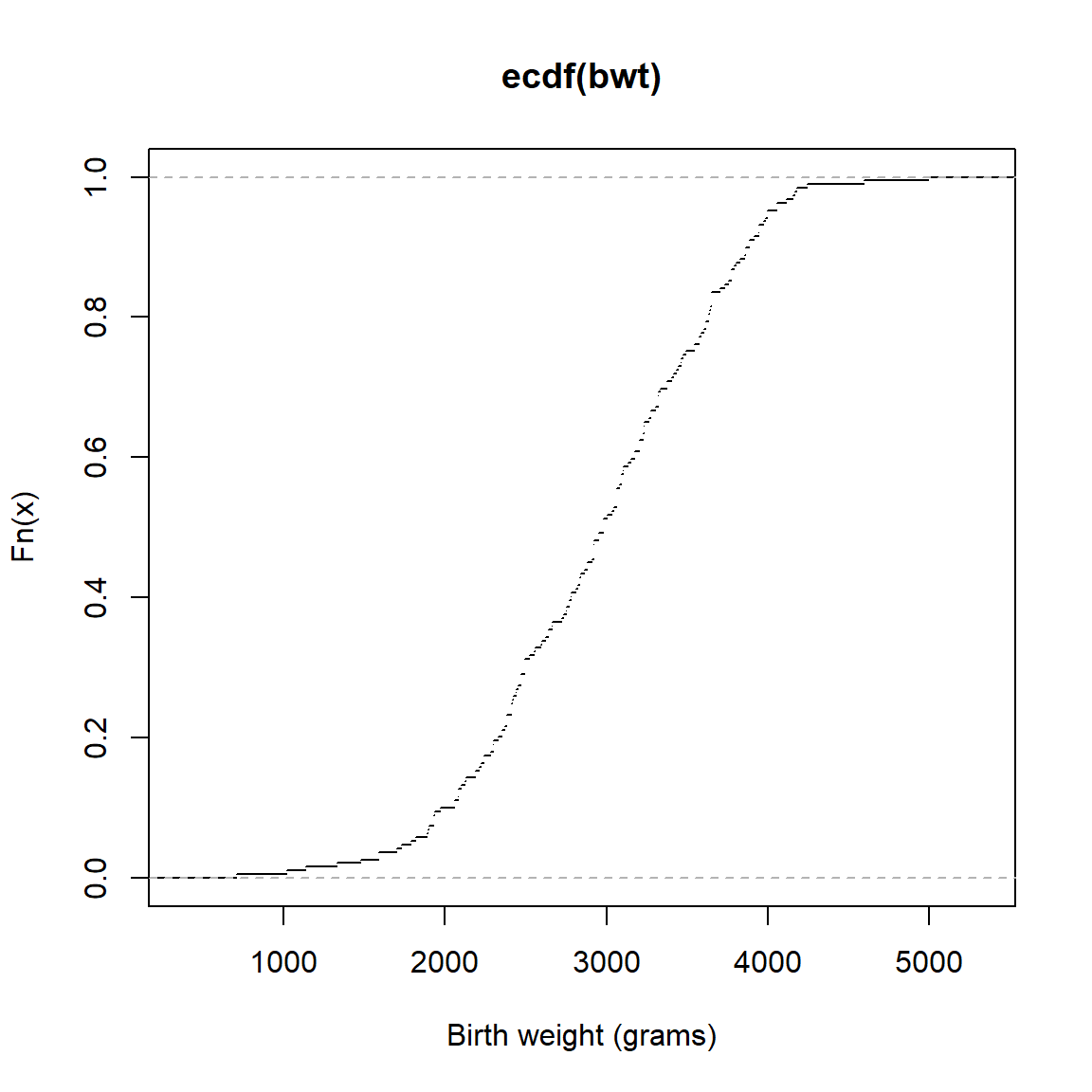• Smoothing
• To estimate a smooth distribution, one might
• Choose from a family of smooth distributions (covered in the previous module)
• Choose from a mixture of smooth distributions (covered in the previous module)
• Use a smoothing kernel (the topic of this module)

### Kernel methods for estimation of smooth distributions

Let’s return briefly to previous module. In the previous module, we considered the birth weight data. We defined the eCDF in the following way: $\hat{F}(x) = \frac{\text{# birth weights} \leq x}{\text{# infants in dataset}}$ Let’s reexpress the eCDF. Let $$\text{bwt}_i$$ denote the $$i^{th}$$ birth weight in the data frame. Define $1(\text{bwt}_i \leq x) = \left\{\begin{array}{ll}1 & \text{bwt}_i \leq x \\ 0 & \text{otherwise} \end{array} \right.$ This is often called the stair step function because of it’s shape. Here we use $$\text{bwt}_i=3000$$ and plot the function.

f <- function(x,bwt) 1*(bwt <= x)
curve(f(x, 3000),2000,4000,n=5000)  #If birth weight = 3000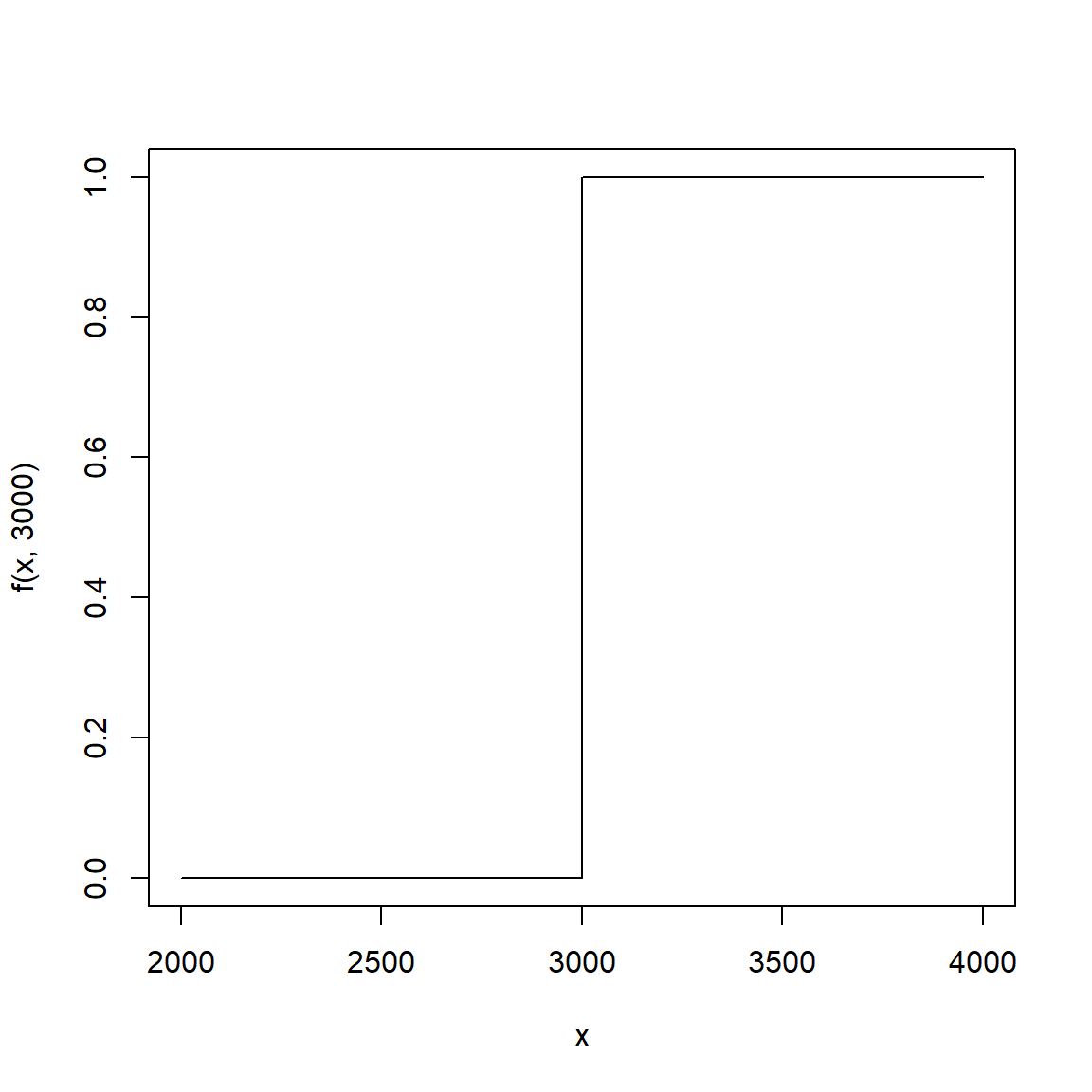In words, stair step function is 1 when the $$i^{th}$$ birth weight is less than $$x$$ and 0 when it is more than $$x$$. To calculate the number of observations in the data frame that have a birth weight less than $$x=2000$$ grams, we can sum over every birth weight in the data frame. \begin{aligned} \text{# birth weights} \leq 2000 & = 1(\text{bwt}_1 \leq 2000) + 1(\text{bwt}_2 \leq 2000) + \dots + 1(\text{bwt}_n \leq 2000) \\ & = \sum_{i=1}^n 1(\text{bwt}_i \leq 2000) \end{aligned} To get the empirical proportion, we can divide by the number of observations in the data frame, typically denoted with $$n$$ or $$N$$.

In general, for any candidate birth weight, $$x$$, the eCDF is $\hat{F}(x) = \sum_{i=1} \frac{1}{n} 1(\text{bwt}_i \leq x)$ Because $$1(\text{bwt}_i \leq x)$$ is a stair-step, the eCDF is also stair-steppy, with abrupt jumps.

One strategy for creating a smooth estimate of the CDF is to replace the stair step function with a smooth alternative. There are lots of options. (See here (link).)

For example, consider replacing the stair step function with the Gaussian CDF centered at $$\text{bwt}_i$$.

f <- function(x,bwt) 1*(bwt <= x)
curve(f(x, 3000),2000,4000,n=5000)  #If birth weight = 3000
g <- function(x,bwt,s) pnorm(x,bwt,s)
curve(g(x, 3000, 100), add=TRUE, lwd = 5, col = "red")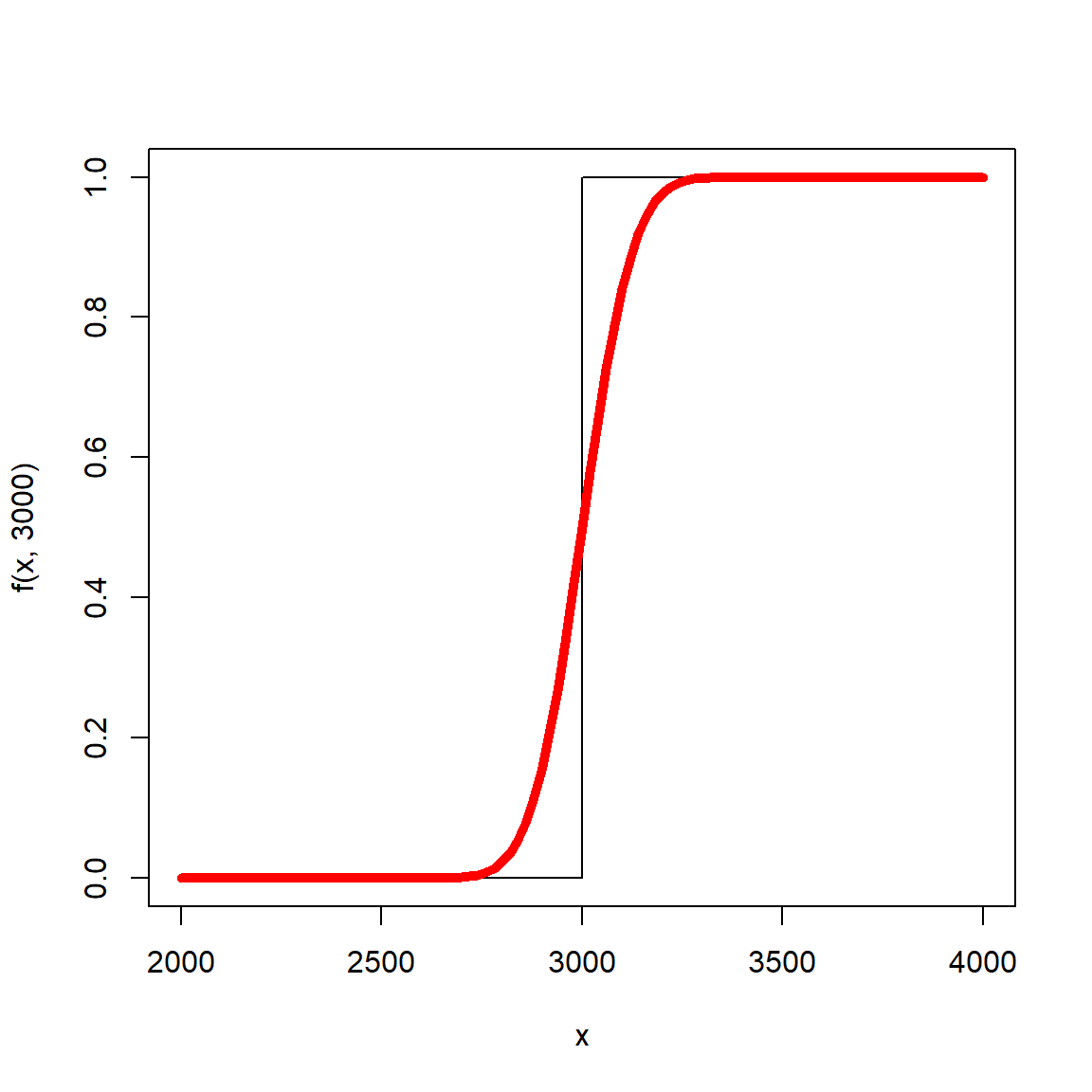The resulting estimate of the distribution function is $\hat{F}(x) = \sum_{i=1} \frac{1}{n} \Phi(x,\text{bwt}_i, s)$

Fhat <- function(x,s) rowMeans(outer(x,bwt,function(a,b) pnorm(a,b,s)))
plot(ecdf(bwt),do.points = FALSE)
legend("left", legend = c("eCDF","Gaussian"), col = c("black","red"), lwd = 3, bty = "n")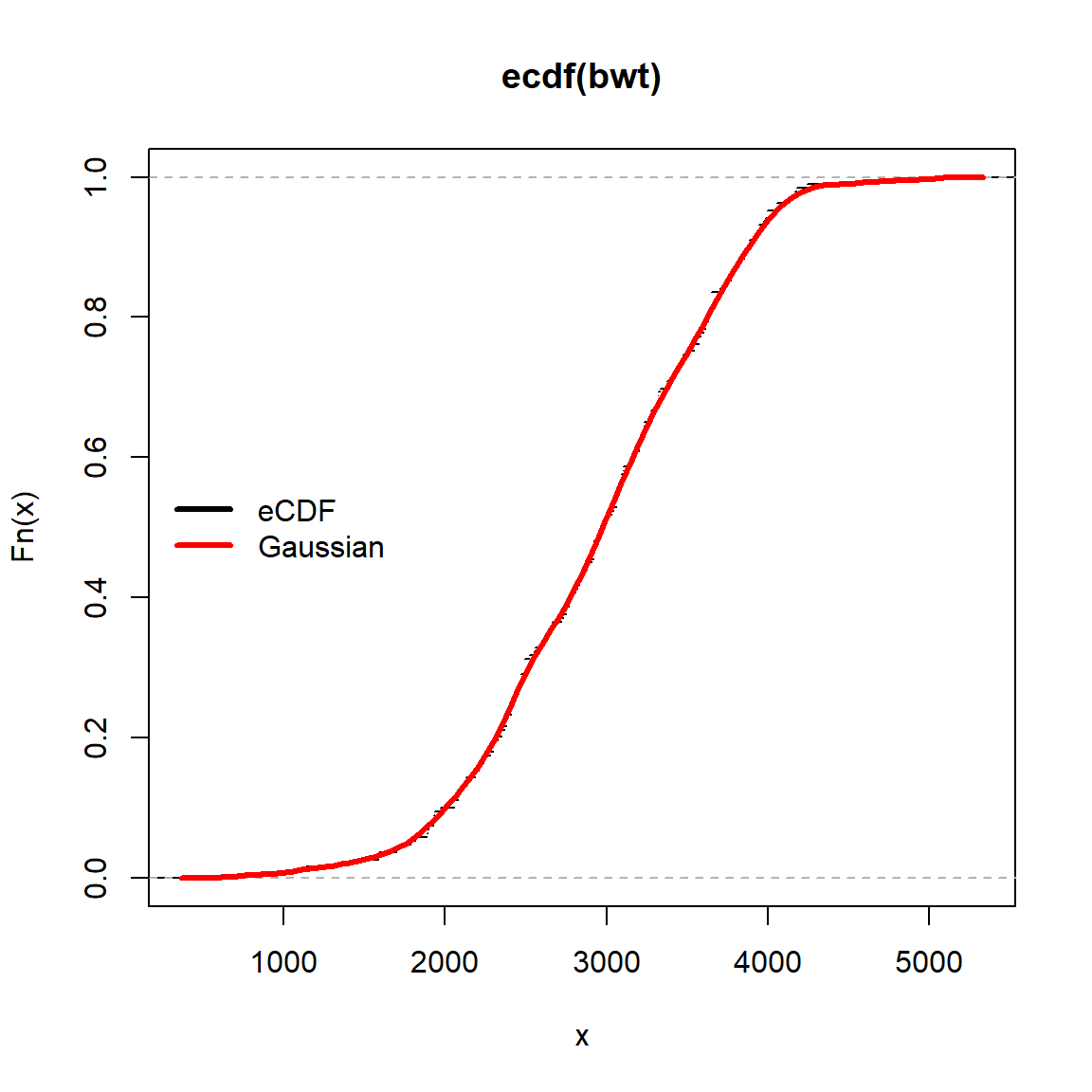The degree of smoothing is controlled by the parameter s. We can increase or decrease the degree of smoothing by increasing or decreasing s.

plot(ecdf(bwt),do.points = FALSE)
legend("left", legend = c("eCDF","Gaussian (s=100)","Gaussian (s=300)"), col = c("black","red","green"), lwd = 3, bty = "n")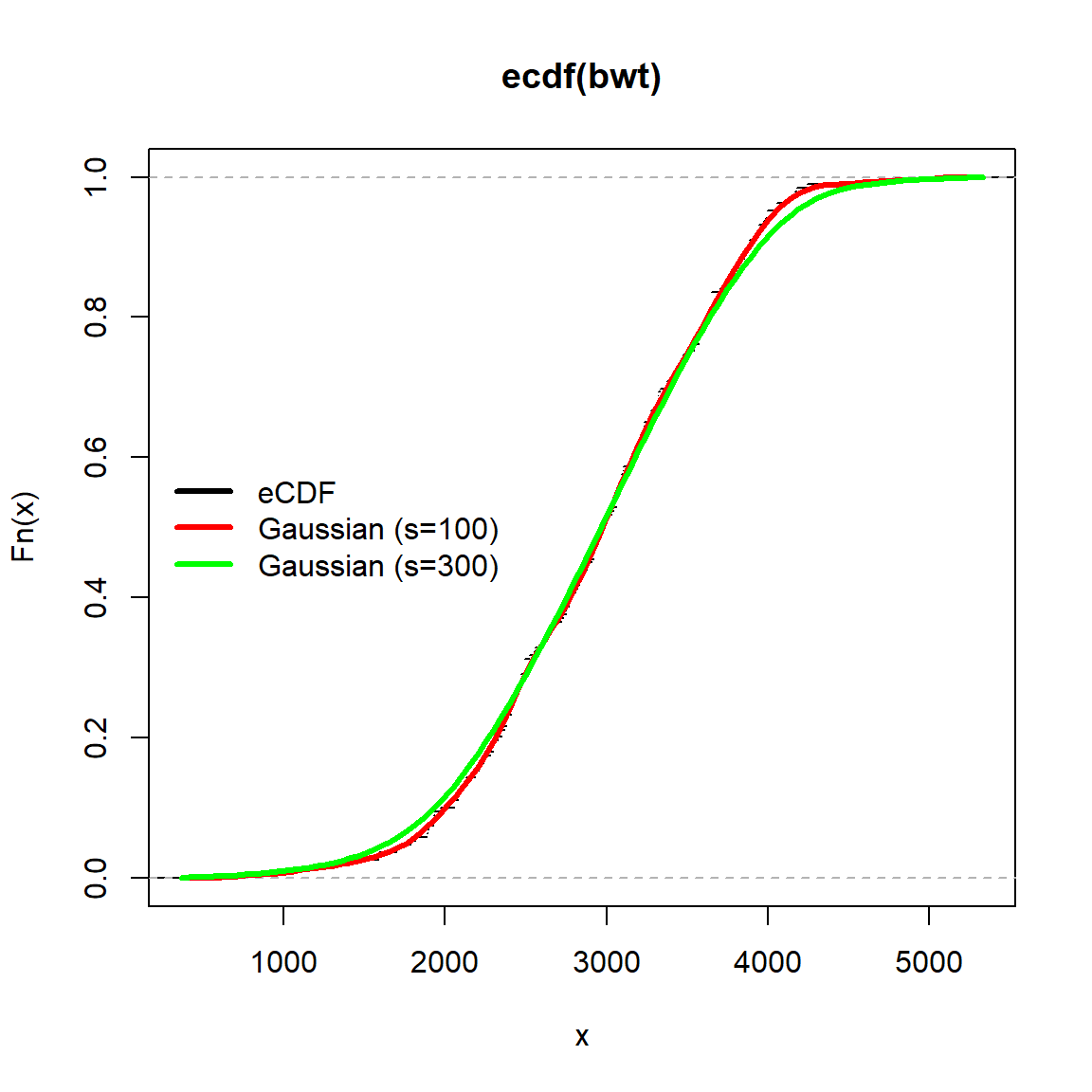This type of estimation is known as kernel smoothing or kernel density estimation. The kernel refers to the specific function selected to replace the stair step function.

In the interactive calculator, your will explore 6 different kernels. Navigate to Birthweight KCDF (link).

In the calculator, you will see the standard eCDF (in black) and the stair step function (in red). A representative kernel function is shown for bwt=3000. As you click on different kernels, you’ll see how the estimate of distribution function changes. You’ll also see how kernel function changes. Also note how the kernels and resulting estimates of the distribution function change as s, the smoothing parameter, changes.

Assessment

1. For each kernel, find a value of s that generates a good estimate of the distribution function. Create an image of the estimated CDF for each kernel. (You can hide the representative kernel function by clicking the circle in line 15 of the calculator.)
2. How did you decide which values of s were better or worse?
3. Using the Gaussian kernel, estimate the distribution function of age. Create a plot in which the eCDF of age is overlaid with the smooth kernel estimate.
4. Using your estimated distribution function, generated an estimate for $$P(\text{age} \leq 25)$$.
5. What are the similarities between kernel smoothing and mixture distributions?